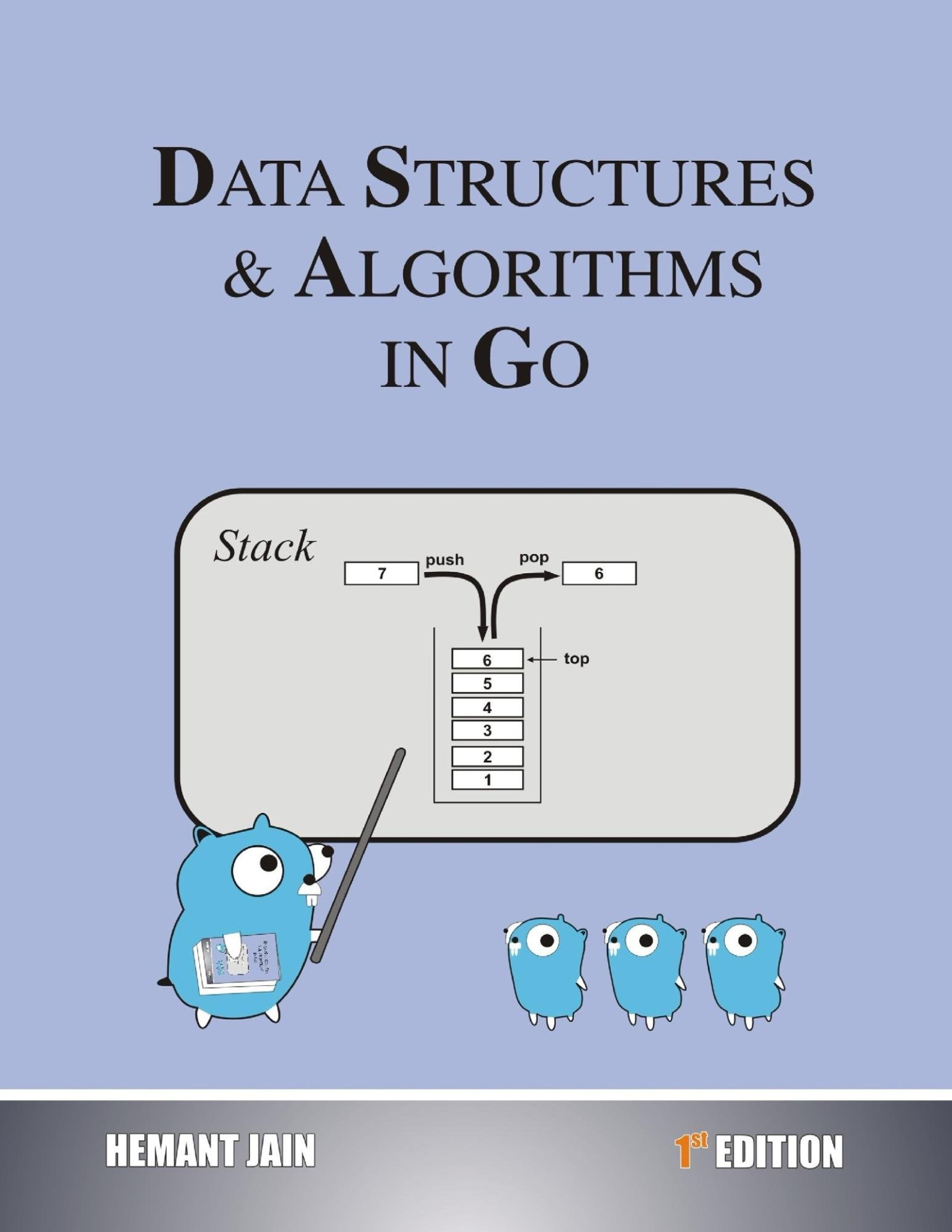### Data Structures & Algorithms In Go

Posted by jack_miller | Published a year agoWith 8 ratings

#### Purchased At: \$20

"Problem Solving in Data Structures & Algorithms" is a series of books about the usage of Data Structures and Algorithms in computer programming. The book is

easy to follow and is written for interview preparation point of view. In various books, the examples are solved in various languages like Go, C, C++, Java, C#,

Python, VB, JavaScript and PHP.

GitHub Repositories

https://github.com/Hemant-Jain-Author

Book’s Composition

This book is designed for interviews so in Chapter 0, various preparation plans are proposed.
Then in chapters 1, a brief introduction of the programming language and concept of recursion is explained. A number of problems based on recursion and array are

explained.

Then in the coming chapter, we will be looking into complexity analysis. Then we will be looking into Sorting & Searching techniques.

Then will look into the various data structures and their algorithms. We will be looking into a Linked List, Stack, Queue, Trees, Heap, Hash Table and Graphs.

Then we will be looking into algorithm analysis, we will be looking into Brute Force algorithms, Greedy algorithms, Divide & Conquer algorithms, Dynamic Programming,

and Backtracking.

In the end, we will be looking into System Design, which will give a systematic approach for solving the design problems in an Interview.

Table of Contents

Chapter 0: How to use this book.

Chapter 1: Introduction - Programming Overview

Chapter 2: Algorithms Analysis

Chapter 3: Approach to solve algorithm design problems

Chapter 4: Abstract Data Type

Chapter 5: Searching

Chapter 6: Sorting

Chapter 7: Linked List

Chapter 8: Stack

Chapter 9: Queue

Chapter 10: Tree

Chapter 11: Priority Queue

Chapter 12: Hash-Table

Chapter 13: Graphs

Chapter 14: String Algorithms

Chapter 15: Algorithm Design Techniques

Chapter 16: Brute Force Algorithm

Chapter 17: Greedy Algorithm

Chapter 18: Divide & Conquer

Chapter 19: Dynamic Programming

Chapter 20: Backtracking

Chapter 21: Complexity Theory

Chapter 22: Interview Strategy

Chapter 23: System Design